# Claes Johnson and the Schwarzschild Equation

Claes Johnson is a Swedish mathematician who, among other things, blogs about climate. His expertise in mathematics doesn’t prevent him from coming to conclusions about climate science that are nonsensical. His mathematical knowledge simply makes it more difficult for non-experts to identify where his analyses go wrong.

Today, I’ll comment on his 2015 essay, Unphysical Schwarzschild vs Physical Model for Radiative Transfer.

This subject is rather technical, and may be understandable only to a limited audience.

One of the main tools that climate scientists use to help analyze planetary temperature are radiative transfer equations which describe how electromagnetic radiation propagates through a medium like the atmosphere. The most important such equation is Schwartzchild’s equation for propagation of thermal radiation. Schwarzchild’s equation has been widely used in engineering and scientific contexts for over a century, and is not limited to applications in climate science.

Johnson’s essay attempts to compare the Shwarzchild equation to another way (of his own devising) for calculating radiation propagation. He finds that the results are different, and interprets this as indicating a flaw in the Schwarzchild equation. He also makes a frivolous claim that the Schwarzchild equation violates the Second Law of Thermodynamics.

Unfortunately, both of the results which Johnson compares are calculated based on incorrect starting equations. So, his comparison is meaningless, and none of his conclusions are justified.

## Misstatement of the Schwarzchild equation

For some reason, Johnson analyzes an equation which he calls the Schwarzchild equation, but which doesn’t match the equation that others call the Schwarzchild equation.

In particular, Johnson’s essay claims that the following is the Schwarzchild equation:

(1)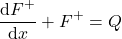(2)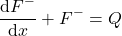where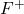is the upward flux of thermal radiation,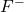is the downward flux of thermal radiation,

(3)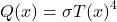and where Johnson seems to assume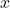is a coordinate corresponding to altitude.

The actual Schwarzchild equation is superficially similar to what Johnson offered, but is different in multiple important ways:

• The standard Schwarzchild equation applies only to a single wavelength,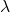, while Johnson seems to be offering an equation that covers all wavelengths.
• Johnson treats his equation as if it is an equation for flux. Yet, the standard equation is an equation for intensity. The difference is that intensity relates to the intensity of radiation propagating in a single direction, while flux relates to the power crossing a plan perpendicular to some direction, but with the radiation propagating in a variety of directions. (There are “two-stream” approximate equations for radiative flux, but such equations are approximations, are not the rigorous Schwarzchild equation, and don’t match the equations that Johnson offers.)
• To calculate the radiation flux at a particular altitude, one needs to solve the standard Schwarzchild equation for all directions (relative to vertical), and sum results over those different directions. The analysis Johnson offers does not do this.
• The standard equation defines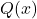differently than Johnson does. In particular, it uses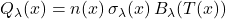where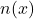is the number density of absorbing molecules,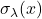is the absorption cross-section of each molecule at wavelengthat position, and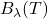is the Planck function giving the emission spectrum of a black-body at temperature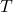at wavelength• The term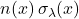corresponds to the emissivity/absorptivity per unit length of the medium–a concept that Johnson entirely omits from his version of the equation. (To offer Johnson the benefit of the doubt, it’s possible to hide this factor if the coordinateis defined in a way that makes this factor implicit. However, this would make the coordinateimplicitly dependent on the wavelength,, as well as on the propagation angle relative to vertical.)

Some readers might be tempted to assert, “Well, all you need to do is sum the standard equation over all wavelengths, and over all directions, and you’ll get Johnson’s version of the Schwarzchild equation.” Unfortunately, that is simply not true. I have solved the standard Schwarzchild equation (under the assumption of a parallel-plane geometry), and the results do not match those naive expectations.

There does not appear to even be any simplified scenario (e.g., absorption being independent of wavelength) which would lead to Johnson’s equations being equivalent to the rigorous Schwarzchild equation.

Sometimes various simplifying approximations are used to evaluation radiation propagation. I have a number of books on radiative transfer. However, I have been unable to locate any approximate radiation propagation equations that match what Johnson offers. (If you have a reference for such equations, please let me know.)

The equations that Johnson presents as the Schwarzchild equation appear to be simply wrong, a misrepresentation of the equation which everyone else refers to by that name.

The equation Johnson critiques is not the equation that climate scientists use in their modeling (and which engineers use when doing thermal analysis for industrial applications).

## Erroneous alternate model

Johnson offers an alternative propagation model “with a one-stream net-flow model based on” [what Johnson refers to as] “Stefan-Boltzmann’s law in its correct physical form” (but which is more conventionally called the radiative heat transfer equation).

This model is expressed as an integral equation in Johnson’s equation (6).

Unfortunately, this equation is built out of contradicting assumptions. It’s assumed that there is a finite absorptivity per unit length,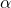. However, it is assumed that the emissivity,, is 1 no matter how thinly the medium is sliced. This latter assumption poses multiple serious problems:

• This assumption about emissivity is mathematically incoherent, and does not allow one to generate any correct integral or differential equation.
• The assumption about emissivity has no correspondence to the properties of any real optical medium (such as the atmosphere).
• The assumption of emissivity violates Kirchhoff’s law of thermal radiation, which states that emissivity must equal absorptivity.

I realize that climate skeptics sometimes use contorted logic to try to deny Kirchhoff’s law (which directly follows from Einstein’s quantum theory of radiation). But, even without invoking Kirchoff’s law, the other identified problems with Johnson‘s assumptions render equation (6) nonsensical.

## Erroneous comparison

Given that both Johnson’s version of the Schwarzchild equation, and his equation (6), are wrong, Johnson’s comparison of what happens when he tries to solve these two models is meaningless.

## Alleged violation of the Second Law of Thermodynamics

Johnson writes “Schwarzschild’s equations (1-2) are supposed to express conservation of upward and downward heat fluxes…” This assertion is wrong in multiple respects:

• The equations are about radiation fluxes, not heat fluxes. In thermodynamics, the term “heat” is a technical term which means “net energy transfer resulting from a temperature difference.” The word “net” is important. The term “heat” refers only to the net result when both fluxes are accounted for, i.e., the term “heat” refers to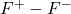. It does not refer toorindividually.
• Radiation fluxes are not necessarily conserved when propagating through an absorbing/emitting medium. Only the total heat flow is conserved. That heat flow is a sum of the radiative heat flow,, and the non-radiative (e.g., convective) heat flow,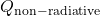. The Schwarzchild equation is fully consistent with the presence of convection, though Johnson seems to be analyzing a purely radiative scenario.

Johnson asserts “Schwarzschild’s two-stream model is unphysical in the sense that the gross downward fluxis directed against the temperature gradient and thus violates the 2nd law of thermodynamics.”

However, the Second Law of Thermodynamics relates to heat flow, andis not a heat flow. As Wikipedia says about this:

“Heat can never pass from a colder to a warmer body without some other change, connected therewith, occurring at the same time.”

The statement by Clausius uses the concept of ‘passage of heat’. As is usual in thermodynamic discussions, this means ‘net transfer of energy as heat’, and does not refer to contributory transfers one way and the other.

So, Johnson is wrong about there being a violation of the Second Law.

## Contention that Stefan-Boltzmann law should involve net flux

Johnson suggests that the “correct form” of the Stefan-Boltzmann law is

(4)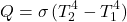as the net radiative heat transfer radiative between two blackbodies of temperature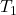and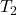with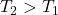, “in accordance with the 2nd law with heat transfer from warm to cold (but not the other way).”

While Johnson offers the correct formula for radiative heat transfer between two black-bodies, his insistence that this is the “correct” form of the Stefan-Boltzmann law is both contrary to standard usage, and ultimately, pointless.

Under most circumstances only the net energy exchange results in physically measurable consequences. (That’s not true under all circumstances, but is generally true for purposes of thermodynamics.) Thus, on philosophical grounds, one might argue that only the net energy exchange is “real” and unidirectional flows of thermal radiation ought to be regarded as in some sense unreal.

If we adopt that position, then unidirectional flows of thermal radiation would be regarded simply a computational trick—a useful tool for calculating the physically meaningful net energy exchange results.

Yet, whether those unidirectional flows are “real” or a simply a “computational trick” doesn’t matter — in either case, the computed observable effects will always be the same, provided one does the calculations correctly.

Johnson finds a difference, but only because he does his calculations incorrectly.

This argument that the Stefan-Boltzmann law should refer to the net radiative energy exchange, rather than the unidirectional radiation emitted, is a silly, pointless argument.

## Another Johnson essay about alleged Schwarzchild equation

Johnson wrote another essay in 2022, Unphysical Schwarzschild Equation for Heat Transfer by Conduction. This essay analyzes a completely fictitious equation which Johnson refers to as the “Schwarzschild Equation for Heat Transfer by Conduction.”

For this essay, Johnson simply made up an incorrect equation (an equation which is not used or endorsed by anybody) and found that his invented equation doesn’t work.

Johnson seems to think his invented equation is somehow analogous to the actual Schwarzchild equation for radiation propagation. However, Johnson shows no signs of understanding the standard Schwarzchild equation. He hasn’t shown evidence that he knows what that equation is, let alone showing any sign of understanding why it is tructured the way it is, or how it functions. So, there is no reason to believe that Johnson is capable of constructing an “analogous equation” which is in any way meaningful.